# Locally convex topology

(diff) ← Older revision | Latest revision (diff) | Newer revision → (diff)
A (not necessarily Hausdorff) topologyon a real or complex topological vector spacethat has a basis consisting of convex sets and is such that the linear operations inare continuous with respect to. A locally convex topologyon a vector spaceis defined analytically by a family of semi-norms (cf. Semi-norm)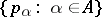as the topology with basis of neighbourhoods of zero consisting of the sets of the form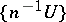, whereruns through the natural numbers andis the family of all finite intersections of the sets of the form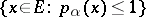,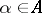; such a family of semi-norms is said to be a generator foror to generate. The topology induced by a given locally convex topology on a vector subspace, the quotient topology on a quotient space and the topology of a product of locally convex topologies, are also locally convex topologies. A topologyon a topological vector spaceis a locally convex topology if and only ifis the topology of uniform convergence on equicontinuous subsets of the adjoint space.
Letand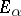,, be vector spaces overor, let(respectively) be a linear mapping ofinto(respectively, ofinto) and let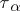be a locally convex topology on,. The weakest topology onfor which allare continuous mappings ofintois called the projective topology onwith respect to the family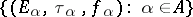. The projective topology is a locally convex topology. In particular, the least upper bound of a family of locally convex topologies on a given vector space, the induced topology on a subspace and the topology of a product of locally convex topologies are projective topologies (and therefore locally convex topologies). The strongest locally convex topology onwith respect to which all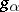,, are continuous mappings of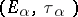intois called the inductive topology onwith respect to the family. In particular, the quotient topology of a given locally convex topology and the topology of a direct sum of locally convex topologies are inductive topologies (and therefore locally convex topologies). The concepts of projective and inductive locally convex topologies make it possible to define the operations of projective and inductive limits in the category of locally convex spaces and their linear mappings.